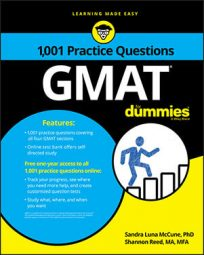##### GMATThe GMAT Quantitative section consists of a mix of Problem Solving and Data Sufficiency questions (37 in total), which must be completed in 75 minutes. (The specific directions for each type of question will be presented each time they appear.)

Problem Solving questions are multiple-choice questions with five answer choice options. Each Data Sufficiency question poses a question followed by two statements. Your task is to evaluate the statements to determine at what point there is or is not sufficient information to answer the question. Unlike the Problem Solving questions, you do not actually have to answer the question posed. Instead, you select one of five fixed answer choices that offer different options about the sufficiency of the information provided in the two statements.

The five answer choices are exactly the same and in the same order for each Data Sufficiency question.

## Quantitative: Problem Solving

• Be prepared to use your knowledge of basic math, probability and statistics, algebra, geometry, and problem solving.
• Memorize formula and other common math knowledge beforehand.
• Write expressions and draw figures on the erasable notepad correctly.
• Avoid making arithmetic or algebra mistakes.
• Check to make sure you didn't overlook something when formulating an equation.
• Eliminate answer choices that don't make sense.
• If a question is taking too much time, make a strategic guess and move on.

## Quantitative: Data Sufficiency

• Memorize the five fixed answer choices so you don't have to refer to them.
• Read carefully to know exactly what the question posed is asking.
• Avoid making unwarranted assumptions, such as assuming a four-sided figure is a rectangle.
• Be sure to check whether the second statement is sufficient when the first statement is determined to be sufficient.
• When the question posed asks for the value of a quantity, decide given information is sufficient only if exactly one numerical value for the quantity can be determined.
• When the question posed is a yes or no question, decide given information is sufficient only if a definite yes or no answer is possible.
• Don't work out solutions to the question posed unless you can't decide sufficiency without doing so.
• If a question is taking too much time, make a strategic guess and move on.